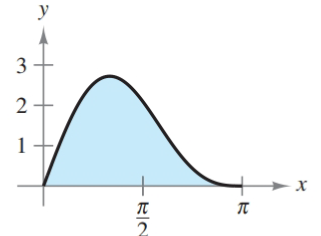Chapter 8.5, Problem 52E### Calculus: An Applied Approach (Min...

10th Edition
Ron Larson
ISBN: 9781305860919

#### Solutions

Chapter
Section### Calculus: An Applied Approach (Min...

10th Edition
Ron Larson
ISBN: 9781305860919
Textbook Problem
1 views

# Finding Area by Integration In Exercises 47-52, find the area of the region. See Example 7. y = 2 sin x + sin 2 xTo determine

To calculate: The area bounded by the graph of y=2sinx+sin2x.

Explanation

Given Information:

The provided graph of y=2sinx+sin2x can be shown as:

Formula used:

The formula of definite integral of absinnudu.

absinnudu=[cosnun]ab

Here, n is the any number.

Calculation:

From provided diagram, the interval [a,b] will be [0, π]. Therefore, area bounded by graph of y=2sinx+sin2x can be calculated as,

0π(2sinx+sin2x)dx=20πsinxdx+0πsin2xdx

Integrate with the help of above integration formulae

### Still sussing out bartleby?

Check out a sample textbook solution.

See a sample solution

#### The Solution to Your Study Problems

Bartleby provides explanations to thousands of textbook problems written by our experts, many with advanced degrees!

Get Started

#### 272/3

Applied Calculus for the Managerial, Life, and Social Sciences: A Brief Approach

#### In problems 37-48, compute and simplify so that only positive exponents remain. 48.

Mathematical Applications for the Management, Life, and Social Sciences

#### What is the primary factor that determines when it is time to change phases?

Research Methods for the Behavioral Sciences (MindTap Course List)